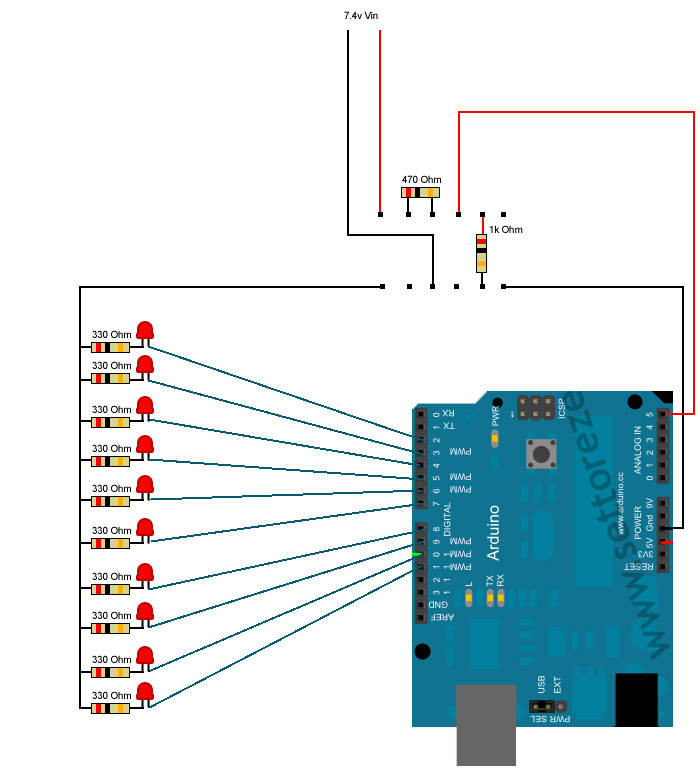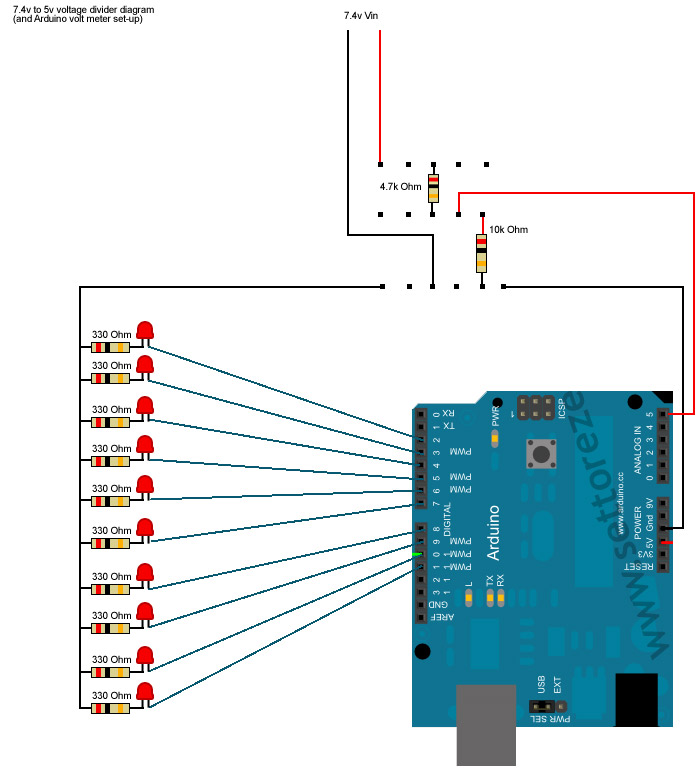# Voltage Divider & Voltmeter help

Okay, then I stand by my recommendation to change the resistor values so that at 8.4V you have 5V at the resistor junction.

5V = (8.4V x 10000)/(x + 10000)

solve for x.

Hi, I have a problem also with a arduino voltmeter/ammeter that is part of a power supply I made. I have written all the code connected it up and it reads the volts and amps pretty accurately on an lcd. My problem is when i connect a load to the power supply the voltage drop shown on the lcd is no where near what it reads on a multimeter. ie. if I set the voltage to say 6.00 volts connect a 3.9ohm load the lcd shows 5.8 volts where as the multimeter shows something around 5.00 volts. and doesn't drop much more with bigger loads either. Does anyone know why this might be?

Mostl likely a math error in your calculation from the analogRead to the voltage you display. Maybe mismatched variable types?

Can't really say without seeing your code tho.

6/3.9 = 1.53846 Amp, is that being displayed correctly?

thought the problem might be electrical but ill attatch the code for you to look at.

but I get about 1.1 amps when i do the maths there so its a little out.

cheers

``````#include
LiquidCrystal lcd(13,12,11,10,9,8);

// These constants won't change:
const int analogPin = A4;    // pin that the sensor is attached to
const int ledPin = 6;       // pin that the LED is attached to
const int threshold = 365;   // an arbitrary threshold level that's in the range of the analog input

int analoginput0 = 3;    //analoge pin 2
int analoginput1 = 4;    //analoge pin 5
float vout1 = 0.0;
float vout2 = 0.0;
int value1 = 0;
int value2 = 0;
float R1 = 5100.0;    // !! resistance of R1 !!
float R2 = 2193.0;     // !! resistance of R2 !!
float vin1 = 0.0;
float vin2 = 0.0;

void setup(){
lcd.begin (16,2);
lcd.setCursor (5,0);
lcd.print ("G'day");
delay (1000);
lcd.setCursor (6,1);
lcd.print ("Mate");
delay (2000);

// initialize the LED pin as an output:
pinMode(ledPin, OUTPUT);
// initialize serial communications:
Serial.begin(9600);

// declaration of pin modes
pinMode(analoginput0, INPUT);
pinMode(analoginput1, INPUT);
lcd.begin(16, 2);

}

void loop(){
// read the value on analog input
value1 = analogRead(analoginput0);   //voltage value
vout1 = (value1 * 4.555)/1023.0;
vin1 = vout1 / (R2/(R1+R2));

value2 = analogRead(analoginput1);    //Amp output
vout2 = (value2 * 4.555)/1023.0;    //4.555=voltage threshold (1023=4.555V);
vin2 = vout2*2;  //vin2 = value2;

lcd.setCursor(12,0);
lcd.print("  ");
lcd.setCursor(8,0);
lcd.print(vin1+0.1);
lcd.setCursor(8,1);
lcd.print(vin2);

lcd.setCursor(0,0);
lcd.print("Volts");

lcd.setCursor(0,1);
lcd.print("Amps");

// read the value of the potentiometer:
int analogValue = analogRead(analogPin);

// if the analog value is high enough, sound alarm:
// if (analogValue > threshold)

if (vin2 > 3.2)      // Set amp alarm value
{
digitalWrite;     //(ledPin, HIGH); //ledpin for led
tone(7, 3);
delay(100);
}
else{
digitalWrite(ledPin,LOW);
noTone(7);
}

delay(100);
}
``````

(code symbols added by moderator)

Why is your threshold voltage 4.555 and not 5.0? Power supply is a little low?

vout = readvalue * 4.55/1023, okay

vout = vin R2/(R1+R2 ) with R1 connected to Vin and R2 connected to gnd vout(R1+R2)/R2 = vin , okay

This seems odd: value2 = analogRead(analoginput1); //Amp output vout2 = (value2 * 4.555)/1023.0; //4.555=voltage threshold (1023=4.555V); vin2 = vout2*2; //vin2 = value2; <<< where does the 2 come from? 0.5 ohm shunt to ground or something?

The 4.555 is the voltage I got when I measured the analogue input variable power supply and multimeter, so 1023 is reached at roughly 4.555 volts not 5 volts exactly, unless its the multimeter telling me different.

and I think i just added the *2 in there just to make the amps close to what it is ment to be. But yes i got a 0.5 ohm shunt on the ground rail.

Im no coding expert and just improvising where i can.

thanks for the help though.

What does the +5V pin measure? The 4.555V is a bit low, maybe your meter is off? All measurements are relitave to the voltage on the +5V pin.

xl97: So I will change out the resistors to be 4.7k & 10k respectively.. and lets me keep the draw form the 'meter' low...

but why @ a stable 7.4v from the PSU am I getting readings of 1023 from +5v - +7.4v??

My guess is that either the resistors you are using are not really 470 ohms and 1K (e.g. the one you think is 1K is actually 10k, or the one you think is 470 ohms is actually 47 ohms), or you are using one of those breadboards that has a break in the middle of the 5v and gnd rails and you haven't taken account of that break (so the 1k resistor is not connected to ground).

Yeh the 4.55 might be a bit low but at the end of the day i have to calibrate it to something and thats the reading i get @ 1023, its really no big deal i can change it to 5 but is less accurate, according to my meter. But its doesn't have anything to do with the voltage drop issue, or lack of it should i say.

ack!.. you thread stealer! =)

anyways..

@ CR-

5V = (8.4V x 10000)/(x + 10000)

should be:

5v = (8.4v x 10000) / (6800 + 10000)

right?

Im still a bit unclear that even with the other resistors in place for a the 7.4v setting.. 'why' it didnt represent a 7.4v 'value' in the serial print?

I mean it was steady 1023 from +5v up to 7.4v..??

I understand why change the resistors.. (since in the end I'll be using 2 x 3.7v li-ions)..

but what was wrong with it currently.. at the 7.4v??

In my mind.. changing out these resistors will do NOTHING for me? (but are only to be put in place because in the end I'll be using a 2 x li-ions)

the question (for me) still remains and is unanswered..

WHY with the current set-up of resistors/voltage divder.. and a 7.4v source am I NOT getting any changes in my analogRea() from 5v to 7.4v?

dc42:

xl97: So I will change out the resistors to be 4.7k & 10k respectively.. and lets me keep the draw form the 'meter' low...

but why @ a stable 7.4v from the PSU am I getting readings of 1023 from +5v - +7.4v??

My guess is that either the resistors you are using are not really 470 ohms and 1K (e.g. the one you think is 1K is actually 10k, or the one you think is 470 ohms is actually 47 ohms), or you are using one of those breadboards that has a break in the middle of the 5v and gnd rails and you haven't taken account of that break (so the 1k resistor is not connected to ground).

sorry I missed this post.. (DOH)..

is there a way I can meter my resistors? to accurately find out the values?

or I suppose I could also try to look up the color bands? (always hate that)..

any better way?

ok..

I took BRAND NEW 4.7k Ohm and a 10k Ohm resistor (fresh out of the pack)... and put them in place

4.7k in between the +Vin and +Vout

the 10k between + and the - (same as illustrated in the picks.. (but the values changed)

and I 'again' get the same results? once I turn the PSU up.. and I get to +5v.. Im already capped at 1023 analog value??

what the 'heck' am I missing here? lol..

I dont even have the LEDS in the mix or part of the project until I can fix the analog read values to be 'somewhat' near my ranges..??

I mean damn.. I only have 2 wires going to the Arduino!!! LOL GND and A5

some resistors..and two leads going to my variable PSU..

It sounds to me that you do not have the 10K resistor connected as you intend. Can you post a photo of your wiring?

If you disconnect the 4k7 resistor, do you get a stable reading of zero from the analog pin?

How about borrowing that 10K pot from your LM386 project.
Since you’re just experiencing, using a pot will make things a little easier.

Edit – corrected drawingdc42: It sounds to me that you do not have the 10K resistor connected as you intend. Can you post a photo of your wiring?

If you disconnect the 4k7 resistor, do you get a stable reading of zero from the analog pin?

hi-

her is the image I made of it.. (using a breadboard)I'll see if the camera is charged (usually isnt)... and post one up of a 'real pic'..if it'll help.

@runaway_pancake-

I'll grab another 10k post I have laying around to use..

and look at your diagrams.

thanks

@runaway_pancake-

I did as your diagram outlined..and it works 'perfect'...

I even left the meter included when I hooked up the Arduino just to keep an eye-on readings.

SO goes back to.. I must be doing something wrong on the breadboard set-up?

here is a pic:thanks for the help guys!.

Your 4k7 resistor is shorted out because you have both ends in the same row of the breadboard.

got ya!. :) thanks. I have also corrected my diagram to show my error/fixI am now going to go back and change out the resistors to match for 8.4v input.

Thanks to everyone for your help. :D

So you've had 7.4V going into your analog pin all this time? That's not good. Does the chip feel warm when powered up now? If so, you likely blew the pin. And if not, it may be partly damaged and fail on you eventually.

"is there a way I can meter my resistors? to accurately find out the values?" Yes, just measure them with your multimeter. You have gold bands on the resistors? I think that's 10% tolerance (maybe 5%, I'm not sure which). If you know the actual values, you can use that in your math for more accurate results.

I would just add: Ohmmeter your resistors out of circuit, [u]Never[/u]* put an ohmmeter on a live/powered circuit.

(* For your meter's sake.)